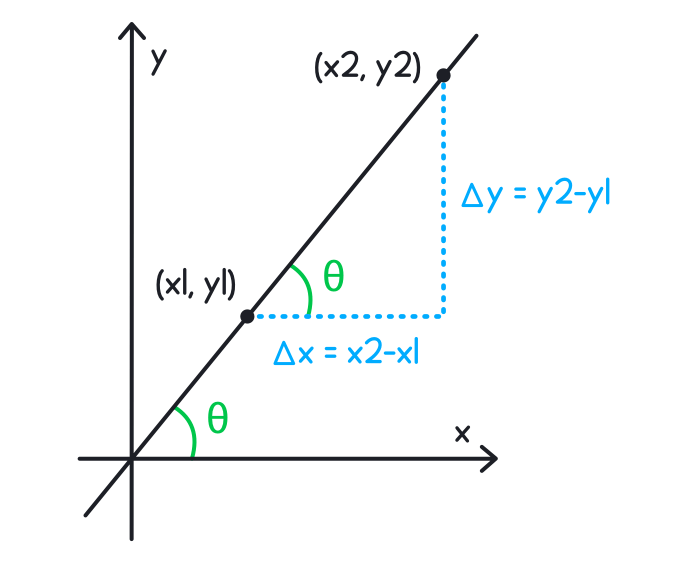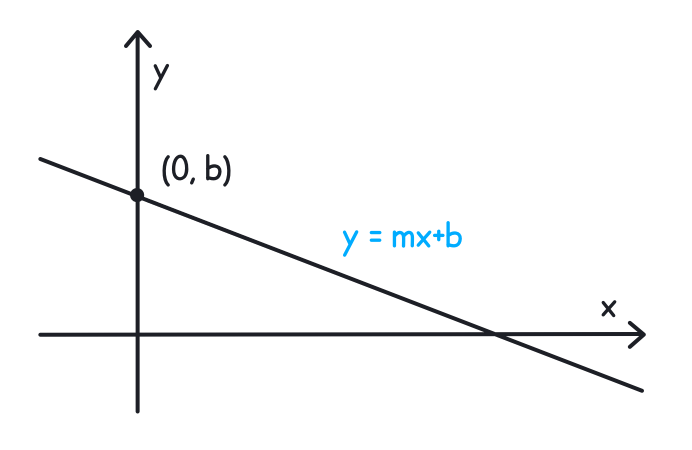### Still have math questions?

Scientific Notation Length Conversion Area Conversion

### Calculatorslope = y2-y1 x2-x1
Point 1
(
,
)
Point 2
(
,
)

Slope =

Intercept form

#### Knowledge

##### Slope

Slope is basically the amount of slant a line has, and can have a positive, negative, zero or undefined value.##### Slope Formula

slope = y2-y1 x2-x1

##### Slope Intercept Form

y = mx + b (with m represents the slope and b represents the intercept ) is the relationship of a straight line.#### Example

What is the slope between (0, 5) and (7, 0)?slope = 0 - 57 - 0 = - 57

Please provide numbers only. Please provide at least two numbers. Please provide 2 to 10 non-zero integer numbers. None